## research papersJOURNAL OFSYNCHROTRONRADIATION
ISSN: 1600-5775
Volume 17| Part 3| May 2010| Pages 386-392

## On the correlation between the X-ray absorption chemical shift and the formal valence state in mixed-valence manganites

aInstituto de Ciencia de Materiales de Aragón, CSIC-Universidad de Zaragoza, Facultad de Ciencias, Pza S. Francisco s/n, 50009 Zaragoza, Spain, and bEuropean Synchrotron Radiation Facility, BP 220, F-38043 Grenoble Cedex 9, France
*Correspondence e-mail: jgr@unizar.es

(Received 21 December 2009; accepted 18 March 2010; online 1 April 2010)

Here the correlation between the chemical shift in X-ray absorption spectroscopy, the geometrical structure and the formal valence state of the Mn atom in mixed-valence manganites are discussed. It is shown that this empirical correlation can be reliably used to determine the formal valence of Mn, using either X-ray absorption spectroscopy or resonant X-ray scattering techniques. The difficulties in obtaining a reliable comparison between experimental XANES spectra and theoretical simulations on an absolute energy scale are revealed. It is concluded that the contributions from the electronic occupation and the local structure to the XANES spectra cannot be separated either experimentally or theoretically. In this way the geometrical and electronic structure of the Mn atom in mixed-valence manganites cannot be described as a bimodal distribution of the formal integer Mn3+ and Mn4+ valence states corresponding to the undoped references.

### 1. Introduction

The concept of ionic valence is widely used to describe the effective number of electrons associated with a given atom within a molecule or solid. This concept applies properly to the case of transition-metal molecular compounds but it starts to lose its meaning when we deal with solid-state materials. The existence of mixed valence in a compound, namely the situation in which the number of electrons assigned is not integral, is rather complex. The question is whether the electronic state of the atom in these formally mixed-valence compounds is genuinely non-integral or whether it can be described as a bimodal distribution of valence states that are integer but different either spatial or temporally. When we refer to mixed-valence transition-metal oxides, d-electronic states are considered to be highly localized giving rise to an integer d occupation. In real materials, however, this picture is only approximated, because the bonding is not 100% ionic. Needless to say that many fascinating phenomena such as high-TC superconductivity in copper oxides, colossal magnetoresistance in manganites or metal-to-insulator phase transitions associated with charge ordering arise from the different distributions of the valence states of the transition-metal (TM) atom. Therefore, it is mandatory to provide a proper description of valence in TM oxides prior to any scientific debate.

One particular case in which a clear definition of mixed valence is especially relevant is charge ordering (CO), a term used to describe the localization of the electrons on the underlying ion lattice in an ordered pattern. In early works, CO was inferred from lattice distortions and magnetic ordering schemes.1 In this way, if two sites for the TM atom are distinguishable either crystallographically or magnetically and the TM is believed to show two different integer oxidation states, the existence of ionic CO would be assumed. Recently, these concepts of valence state and CO have been reconsidered (Subías et al., 1997; Garcia et al., 2001; Garcia & Subías, 2004) within the framework of X-ray absorption spectroscopy (XAS) and resonant X-ray scattering (RXS) experiments. These techniques allow us to determine the valence of the TM atom by means of the chemical shift, which correlates the energy position of the absorption edge with the formal valence. Based on this empirical correlation, XAS experiments have shown that the valence of Mn atoms in mixed-valence manganites is fractional and it cannot be described as a bimodal distribution of two Mn ions with different integer d occupations (Subías et al., 1997; Garcia et al., 2001). Moreover, RXS experiments have concluded that the charge disproportion between the different Mn atoms in the CO phase is generally much less than one electron, each individual valence being then fractional (Garcia & Subías, 2004; Herrero-Martin et al., 2004; Garcia & Subías, 2006; Grenier et al., 2004). However, several works challenge these methods (Ignatov et al., 2001; Chaboy, 2009), casting doubts on the reliability of the aforementioned empirical correlation to determine the valence state of TM atoms in mixed-valence oxides. These criticisms are based on theoretical calculations that intend to separate local structural geometry from electronic occupation.

We will discuss here the information that the experimental methods provide on the valence state of the atoms in a solid. We will mainly focus on XAS and RXS techniques, where the valence can be inferred from the chemical shift. We will comment on the reliability of using this empirical method and on inconsistencies of some interpretations on the basis of the following points: (i) what is the accuracy of the edge-shift method when comparing XAS spectra? (ii) can we use the polarized XAS spectra to determine the valence state? (iii) is it appropriate to artificially distinguish between two chemical shifts, one reflecting the local geometry and the other induced by the electronic occupation? and (iv) can we compare experimental XAS data with theoretical calculations on an absolute energy scale? Finally, we will show through a simple experiment that the XAS spectrum of a `pure' mixed-valence compound, i.e. a water solution of Fe2+ and Fe3+ ions, can be obtained by the weighted addition of the XAS spectra of the two individual ionic species. This contrasts with the case of mixed-valence TM oxides, where the spectrum cannot be obtained as a factorized addition of the spectra of the integer valence compounds.

### 2. Experimental methods to determine the valence state

Formal (or classical) ionic valence is associated with the number of electrons an atom donates (cation) or accepts (anion) when forming a compound. Generally this number is integral and is solely derived from the chemical composition of the compound. In manganese oxides we denote the number as the formal valence state of the Mn atom, which comes from the neutrality of the formula unit, assuming O2− ions. For example, the presence of Ca2+ and La3+ in La0.5Ca0.5MnO3 implies a Mn+3.5 formal valence state, which results from 0.5 × (−3) + 0.5 × (−2) + 3 × (+2) = +3.5. This fractional valence state will represent the charge at the Mn atom in the hypothesis of ideal ionic bonds. However, the effective charge is not known and can vary significantly from this formal valence owing to covalency with O atoms.

Despite the effective charge on an atom being not easily determined, we can use several empirical methods to estimate the formal valence state of an atom. Most of these methods are based on a fingerprint technique, i.e. comparison with reference systems. One of these methods is X-ray photoelectron spectroscopy (XPS). XPS measures the binding energy of a core level. Since the chemical state of an atom alters the binding energy of the deep level, XPS is useful to identify the formal valence state and ligands of an atom by the use of the chemical shift information. In this way the same binding energy is obtained for two compounds containing atoms in the same formal valence state n+ but it is different from the binding energy of a compound where the atoms present a formal valence p+. We would like to emphasize that the specific electronic state of the atom cannot be deduced except for the fact that it shows a strong similarity with other compounds with formally the same valence state. However, we cannot differentiate whether the variation of the binding energy is due to the different electronic charge occupation or whether it comes from the different local structure. Fig. 1shows a classical example, the carbon 1s spectrum of the organic compound ethyl trifluoroacetate, where the binding energies for the different C atoms according to the ligands to which they are connected are shown. It is straightforward to see that we cannot associate an integer valence state with any of the C atoms owing to the covalent character of the organic compounds. However, we can conclude that the charge localized on each of four inequivalent C atoms is different (Gelius et al., 1973).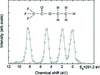Figure 1 XPS spectrum of ethyl trifluoroacetate in the C(1s) range showing the different chemical shifts for the four inequivalent C atoms, taken from Gelius et al. (1973).

Another method to determine the valence of an atom is by use of the bond valence sum (BVS) rule (Brese & O'Keeffe, 1991; Brown, 1991). The application of BVS has been very successful in interpreting crystal structures, and deviations of the calculated valence from the integer formal valence have been deduced in many cases. This method establishes that the valence Vi of an atom i is distributed over all the bonds between atom i and the atoms j of its environment according to the sum of so-called bond valences (bij) of each particular bond. The bond valences bij are a monotonously decreasing function of the interatomic distances dij so the valence of the atom depends on the addition of nearest-neighbour inter­atomic distances, in other words, on the averaged 〈dij〉 distance. To illustrate this fact, Fig. 2shows the correlation between the formal valence state derived from the stoichiometry and the averaged interatomic distance (〈dMn–O〉) in the corresponding MnO6 octahedron for three series of compounds La1–xCaxMnO3 (Subías et al., 1998a, 2002), LaMnO3+δ (Subías et al., 1998b) and La1–xSr1+xMnO4 (Senff et al., 2005; Herrero-Martin et al., 2005). A simple monotonic correlation between the contraction of 〈dMn–O〉 and the increasing of the formal valence is observed. This correlation also holds for systems with different local symmetry. In the La1–xSr1+xMnO4 series the local symmetry around the Mn atom evolves from a strong tetragonal distortion [averaged distances perpendicular and parallel to the tetragonal distortion (c) axis are 1.90 and 2.27 Å, respectively] for x = 0 to an almost regular octahedron [averaged distances perpendicular and parallel to the tetragonal distortion (c) axis are 1.92 and 1.98 Å, respectively] for x = 0.5. From the figure we can infer a non-negligible uncertainty of about 0.02 Å in 〈dMn–O〉 for systems with formally the same valence that corresponds to an error in valence of 0.2 valence units. In any case we can establish that the averaged Mn—O distance in mixed-valence manganites is intermediate between the two end-members beyond the uncertainty of this correlation. This is also in agreement with an intermediate valence state for the Mn atom. Again, the formal valence state is extremely sensitive to the local bond lengths and coordination.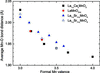Figure 2 Correlation between the formal valence state of the Mn atom and the average of the six Mn—O interatomic distances of the individual MnO6 octahedron in several manganites. Data taken from Subias et al. (1998a, 2002) (black squares), Subias et al. (1998b) (red circles), Senff et al. (2005) (filled blue triangles) and Herrero-Martin et al. (2005) (empty blue triangles).

We concentrate now on the empirical method based on XAS spectroscopy. In this case the energy position of the absorption edge indicates the formal valence state (chemical shift). As a matter of illustration, Fig. 3(a)shows X-ray absorption near-edge structure (XANES) spectra of the La1–xCaxMnO3 series. The absorption edge shifts to higher energies as the formal valence increases following almost a linear dependence (Subías et al., 1997; Garcia et al., 2001). This correlation between the chemical shift and the formal valence state is reported in Fig. 3(b). We would like to note that this method has its own uncertainty. As a matter of illustration, we show (Fig. 3a) the comparison between XANES spectra of LaMnO3 and LaMn0.4Ga0.6O3 compounds, where the local structure of the Mn atoms changes from a tetragonal distorted configuration towards a symmetric octahedron, keeping, however, the same 3+ formal valence (Sanchez et al., 2004, 2006). The differences in the energy position of the absorption edge is within 0.3 eV, which indicates that the valence state for the Mn atom is 3+ within an error of 0.1 valence units in all compounds despite variations in the local structure.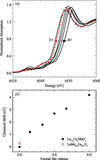Figure 3 (a) Comparison of the experimental XANES spectra through the series La1–xCaxMnO3 (black solid lines; from left to right x = 0, 0.33, 0.5, 0.66 and 1) and LaMn0.4Ga0.6O3 (red closed circles). (b) Correlation between the formal valence state of the Mn atom and the chemical shifts of the Mn K-edge with respect to LaMnO3 in several manganites.

Here, attention must be paid to the fact that the absorption coefficient is not scalar but a tensor and it depends on the polarization of X-rays. Fig. 4shows the polarization dependence for the La1+xSr1–xMnO4 series, where Mn atoms are surrounded by a tetragonal distorted octahedron of O atoms. Averaged distances perpendicular 〈d(Mn–O)⊥〉 and parallel 〈d(Mn–O)||〉 to the tetragonal distortion (c) axis are 1.90 and 2.27 Å (x = 0), 1.92 and 2.08 Å (x = 0.3), and 1.92 and 1.98 Å (x = 0.5), respectively. We observe that the energy position of the absorption edge for incident X-rays polarized parallel to the c axis (||) is shifted to lower energies, according to the longer distances. Moreover, we also observe that, for incident X-ray polarization within the ab plane (⊥c), the energy position of the absorption edge is almost independent of the formal valence state of Mn, in agreement with the slight variation of the Mn—O distances in the ab plane. This comparison suggests that the chemical shift is mainly sensitive to the nearest-neighbours bond distances (Subías et al., 1998a, 2002), as is generally admitted (Glatzel et al., 2006, and references therein), and similar to the BVS rule. Therefore, the empirical correlation between the chemical shift and the formal valence state is only applicable to the non-polarized X-ray absorption coefficient (i.e. the trace of the absorption coefficient tensor) and shall not be extended to its individual tensor components. In any case, variations in the local structure significantly alter the density of states of valence electrons and thus there is an empirical correlation between the formal valence and the chemical shift (Lengeler, 2006).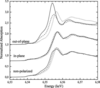Figure 4 Comparison between the Mn K-edge XANES spectra of LaSrMnO4 (solid lines), La1.7Sr0.3MnO4 (dashed lines) and La1.5Sr0.5MnO4 (dotted lines) compounds for the non-polarized, in-plane (electric field vector E parallel to the ab plane) and out-of-plane (electric field vector E parallel to the c axis) polarized configurations.

The controversy arises from the strict interpretation of the formal valence as only an electronic occupation. For example, the formal valence of the Mn atom in La0.5Ca0.5MnO3 is +3.5. This just means that the valence state of the Mn atom in La0.5Ca0.5MnO3 is intermediate between those it shows in LaMnO3 and CaMnO3 if we formally call these valence states for Mn atoms as +3 and +4, respectively. The assumption that Mn is in a 3d3.5 configuration (i.e. the effective atomic charge is 3.5+) cannot be deduced from any of the above-discussed methods. However, all of them prove that the effective charge and the local geometric structure are intimately correlated. Therefore, attempting to separate the contribution of each to the formal valence (or to the chemical shift) does not have physical meaning. From a theoretical point of view, the separation of the contribution from the electronic occupation and that from the local geometry is highly dependent on the method used. We will discuss this point in the following section.

### 3. Limitations of the theory

Theoretical calculations of XANES spectra are usually carried out in the framework of the multiple scattering theory (Natoli & Benfatto, 1986; Benfatto et al., 1986; Ankudinov et al., 1998). We refer here to the CONTINUUM (MXAN) (C. R. Natoli, unpublished), FDMNES (Joly, 2001) and FEFF8 (Rehr & Albers, 2000; see also https://FEFF.phys.washington.edu/ ) codes. In this approach the final-state wavefunction is calculated in terms of scattering of the photoelectron with the atoms surrounding the absorbing one. In other words the wavefunction of the final state corresponds to one electron moving in the potential created by itself and its near neighbouring atoms and it strongly depends on how realistic the description of the potential is for the different atomic clusters. Concerning a theoretical study of the absorption-edge shifts, we discuss here the different constrains of the computations. Present codes calculate the absorption cross section taking a constant potential (the muffin-tin potential when this approach is used) as a reference for the energy of the final-state wavefunction. In other words they do not calculate the energy necessary to promote the core electron to the continuum states. Therefore, theoretical calculations nicely reproduce the energy dependence of the XANES spectrum but they cannot be directly compared with experimental XANES spectra. Since the photo-ionization (binding) energy depends on the valence state, it is necessary to add the binding energy to the chemical shift obtained by the theoretical calculation for a comparison on an absolute energy scale.

The question arises as to whether present codes can compute the binding energy of a core level with enough accuracy to theoretically describe the chemical shifts in XANES spectra. The binding energy of a core level is obtained by calculating the total energies of the ground and the excited states for the atomic cluster and subtracting them. In the particular case of manganites, the total energy of the ground state for the Mn atom is about 2 × 104 eV. The accuracy of the calculations is about 5 × 10−4. This means that the absolute error is about 10 eV. This is much larger than the chemical shift between Mn3+ and Mn4+, approximately 4 eV. Consequently, the conclusions derived from the theoretical studies reported in the literature on the chemical shift (Chaboy, 2009; Nazarenko et al., 2006) must be taken with care. To increase the accuracy of these calculations is at least necessary to perform self-consistent calculations (SCF) of the whole electronic configuration, but this calculus is almost impossible for large clusters from a technical point of view.

Another question is the separate analysis of local geometry and electronic occupation. Some authors attempt it either by changing the electronic occupation and leaving the geometry unaltered (Joly, 2001; Nazarenko et al., 2006), or maintaining the electronic occupation while changing the geometry (Chaboy, 2009). In both cases this is an unfruitful task since the electronic configuration depends on the geometry and the geometry determines the electronic configuration. In fact, as we commented before, SCF potentials are necessary to obtain accurate binding energies but these potentials are highly dependent on the geometry of the atomic cluster. In order to identify the mechanisms that cause the chemical shift in manganites, de Vries et al. (2003) performed ab initio SCF calculations in embedded Mn ions and MnO6 clusters of the 1s ionization as well as the 1s–4p transition energies. They concluded that both effects, geometry and electronic configuration, must be taken into account together to obtain reliable values of either the 1s ionization or the absorption K-edge energies.

### 4. Intermediate valence and charge ordering

Let us now treat in detail the case of mixed-valence compounds that show CO. In these compounds the formal valence state of the Mn atom has been implicitly and explicitly described as a bimodal distribution of Mn3+ and Mn4+ in adequate proportion, xMn3+ + (1 − x)Mn4+. One way to check if this assignation is correct is by means of XAS spectroscopy as we have commented in §2.

XAS directly probes the local atomic structure and thus it can be used to characterize the valence state of an atom. In particular, the XANES spectrum of a compound at the absorption edge of an atom A that appears in two different oxidation states An+ and A(n+1)+ characterizes its valence because of the different energy positions of their absorption edges. In the case of a compound containing a bimodal distribution of An+ and A(n+1)+ ions, the XAS spectrum will be equivalent to the weighted addition of the An+ and A(n+1)+ XAS spectra. This is a corollary of the principles of the XAS spectroscopy, where the spectrum of a sample corresponds to the incoherent addition of the individual photo-excitation processes. As a matter of illustration, Fig. 5shows the experimental Fe K-edge XANES spectrum of a 0.1 M water solution, which contains Fe3+ and Fe2+ ions in a 1:1 ratio, compared with experimental XANES spectra of 0.1 M water solutions of pure Fe3+ and Fe2+ ions, respectively (Benfatto et al., 2002). The agreement between the experimental XANES spectrum of the mixed Fe2+–Fe3+ water solution and the weighted addition of the spectra of both Fe3+ and Fe2+ water solutions is remarkable, as shown in the lower panel of Fig. 5. We note that this agreement extends to the whole XANES spectra, up to 100 eV above the absorption edge. This comparison confirms the reliability of the empirical method based on XANES spectroscopy to identify different ionic species in a compound.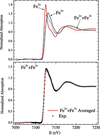Figure 5 Upper panel: experimental XANES spectra at the Fe K-edge of Fe2+ (red solid line), Fe3+ (black solid line) and 50% Fe2+ + 50% Fe3+ (blue dashed line) of 0.1 M solutions in water at pH = 1. Lower panel: comparison of the XANES spectrum of the mixed Fe3+–Fe2+ solution with the weighted addition of the Fe2+ and Fe3+ solution spectra in a 1:1 ratio.

When dealing with mixed-valence manganites, a similar comparison does not work. The XANES spectra of the intermediate mixed-valence manganites cannot be obtained as the weighted sum of the XANES spectra of the formal integer valence compounds (Subías et al., 1997; Garcia et al., 2001; Booth et al., 1998; Bridges et al., 2001). The question is whether XANES spectra for the end-members of the series are appropriate references for each integer valence state since the local geometry around the metal atom in those integer valence compounds is different from that in the mixed-valence ones. We note that the line shape of the XAS spectrum depends on the geometry of the atomic cluster surrounding the absorbing atom, which extends beyond the first coordination shell. For instance, Mn3+ is only surrounded by La atoms in LaMnO3 whereas a hypothetical Mn3+ atom will be surrounded by both La and Ca atoms in La0.5Ca0.5MnO3. However, XANES spectra of several half-doped manganites with different chemical compositions (Garcia et al., 2001) are very similar to each other. The main difference between them comes from the different energy position of the absorption edge whereas the effect of distant shells on the XANES spectra is not significant. Therefore, taking into account that the energy position of the absorption edge mainly depends on the valence state (chemical shift), it is appropriate to carry out this type of comparison if we attend exclusively to the neighbourhood of the rising edge in the XANES spectrum. Fig. 6clearly shows that a bimodal Mn3+–Mn4+ distribution will give a double step at the rising absorption edge that is not observed in the experimental XANES spectrum of La0.5Ca0.5MnO3.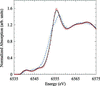Figure 6 Comparison between the experimental high-resolution XANES spectrum at the Mn K-edge of La0.5Ca0.5MnO3 (red open circles) with the weighted sum (50%:50%) of the end-members LaMnO3 and CaMnO3 (blue dashed line). In addition, the weighted sum (50%:50%) of the intermediate La0.67Ca0.33MnO3 and La0.33Ca0.67MnO3 compounds (black solid line) is also shown.

These results discard the presence of a mixture of ionic-like Mn3+ and Mn4+ atoms in the La1–xCaxMnO3 compounds. This is not surprising since the atomic structure around the Mn atoms evolves continuously with doping. Neither neutron nor X-ray diffraction and EXAFS have detected a bimodal distribution of the interatomic distances corresponding to Mn3+ and Mn4+ references. However, the XANES spectrum of La0.5Ca0.5MnO3 can be nicely reproduced by the weighted sum of La0.66Ca0.33MnO3 and La0.33Ca0.66MnO3, whose chemical shift is only about 1 eV (Fig. 6). This shows that the electronic and local structure of Mn atoms in the intermediate compounds is similar to each other so there is limited sensitivity to detect small changes of valence. Similar examples of this limitation are also found in X-ray emission spectroscopy, where the difference between the emission spectra of the reference compounds can be too small to be conclusive (Tyson et al., 1999; Qian et al., 2001). However, this comparison points to two different Mn local environments associated with two different non-integral intermediate valence states.

The same quality of agreement is obtained for the comparison between the experimental XANES spectra of La0.5Ca0.5MnO3 and La0.33Ca0.67MnO3 with their respective averaged sums of the end-members spectra, assuming that the chemical shift is only 2 eV instead of 4 eV, as shown in Figs. 7 and 8, respectively, of Chaboy (2009). The argument used by Chaboy (2009) for such a reduction is to subtract the chemical shift coming from the geometry and the lattice from that originated by the electronic structure. However, this implies that very different Mn3+ (or Mn4+) ions from the point of view of their local environment (and indeed from the point of view of their electronic state) exist for the different intermediate cases. This result contrasts with the LaMn0.1Ga0.9O3 compound. Fig. 7shows the local structure around the formal Mn3+ in this sample that consists of a regular octahedron of O atoms instead of the tetragonal Jahn–Teller distortion but the chemical shift corresponding to this change of the distortion is only 0.4 eV. The fact that the nearest-neighbours interatomic distances and formal valence states are intimately joined is experimentally supported for instance by the XANES of LaMn0.5Mg0.5O3, where the Mn atom shows the same inter­atomic Mn–O distance and the same absorption K-edge energy as CaMnO3, despite their different lattice (Blasco et al., 2004). Finally, a similar procedure applied to the previous given example (Fe3+/Fe2+ in water) will yield absurd conclusions.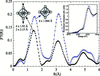Figure 7 Comparison between the modulus of the Fourier transform of the k-weighted EXAFS spectra of LaMnO3 (black closed circles) and LaMn0.1Ga0.9O3 (blue open circles), showing the change in the local structure of the MnO6 octahedron. The inset displays the corresponding normalized XANES spectra at the Mn K-edge.

In spite of the limited sensitivity of the simple analysis of XANES edge-shifts to small changes in the valence state, the strong empirical correlation between the chemical shift and the formal valence has also been very useful to characterize CO compounds by means of RXS (Materlik et al., 1994; Subías et al., 2009; Di Matteo, 2009). This technique profits from the strong enhancement of the intensity of new satellite reflections appearing in the CO phase when the energy of the X-rays is tuned across the TM absorption edge. Resonances appear because the anomalous part of the atomic scattering factors of atoms occupying different i and j crystallographic sites are different from each other. In other words the structure factor for these reflections is F ≃ (fifj), where fi is the atomic anomalous scattering factor of the TM atom at site i. Since the imaginary part of f is proportional to the absorption cross section and the real part is related to the imaginary part by the Kramers–Kronig integral relations, resonances come from the different XANES spectra of the resonant atoms. The differences between the XANES spectra essentially arise from the different energy position of the absorption edge for the resonant atoms at different i and j crystallographic sites, which makes the resonance appear at the absorption edge. In this way it is possible to estimate the chemical shift between the two different resonant atoms. Many RXS papers have focused on CO [we address the reader to our recent review on RXS in TM oxides presented at the XAFS XIV conference (Subías et al., 2009)]. Most of these works have shown that the charge disproportion between the different TM atoms (estimated from the chemical shift) is very small and far from one electron. It is noteworthy that the values given by RXS for the intermediate valence states are related to the chemical shift between the integer formal valence states and thus they are only a formal parameterization of the valence state. Even in this case, any attempt to separate contributions coming from the geometrical structure and the electronic occupation to the origin of the different atomic scattering factors has no physical meaning.

### 5. Conclusions

The occurrence of intrinsically mixed-valence states in TM atoms has modified the consensus relating to the description of their electronic structure. It was generally accepted that TM atoms show an integer valence state, which corresponds to a dn configuration with n integer. The experimental XAS results show that this belief is far from the reality, but they have encouraged theoretical works attempting to demonstrate that these experimental results fail in their interpretation (Ignatov et al., 2001; Chaboy, 2009).

We have critically revised these approaches for disentangling the various mechanisms that affect XANES spectra of TM compounds, and the following conclusions were obtained. First, we have shown that electronic occupation and geometrical structure are intimately connected and an attempt to separate each contribution to the XANES spectrum is not appropriate. Second, it is mandatory to calculate the photo-ionization energy prior in order to make a reliable comparison between experimental XANES and RXS spectra with theoretical calculations on an absolute energy scale. However, at the present status of the theoretical calculations, the computation of the core-hole binding energy is far from the precision required in the analysis of the chemical shift.

Summarizing, the empirical correlation between the chemical shift in XAS and RXS and the formal valence state of TM atoms can be reliably used to describe the electronic state of mixed-valence TM compounds, including charge-ordering phases. We stress that there is enough experimental evidence to support TM atoms in formal mixed-valence compounds, such as manganites, being different from TM atoms in the integer valence reference compounds in both their local structure and their electronic valence state.

### Footnotes

1Almost all the papers on CO before 2000 consider explicitly or implicitly the existence of a bimodal distribution of two integer valence states. As an example, see Radaelli et al. (1997).

### Acknowledgements

This work was supported by the Spanish MICINN FIS2008-03951 project and Diputación General de Aragón (DGA-CAMRADS). Javier Blasco and M. Concepción Sánchez are acknowledged for fruitful discussions and comments. VC thanks the FPU research grant from MICINN. ESRF and beamline BM25 are acknowledged for granting beam time to record XANES spectra of iron water solutions.

### ReferencesJOURNAL OFSYNCHROTRONRADIATION